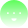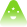Getting Started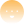Learn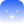Use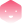Build
learn

# Pool Valuation (NAV)

## Introduction

### What is asset valuation?

Asset valuation is the process of determining the current worth of an asset or portfolio, often expressed as the net asset value (NAV). This valuation is typically required when an asset or portfolio of assets is being sold, or when investors want to enter or exit an existing investment fund or pool. In such cases, the portfolio's value ultimately determines the investment or redemption price.

### Approaches to asset valuation

Depending on the asset class, there may be different approaches to valuing an asset or pool of assets. For assets with a public liquid secondary market, such as stocks, bonds, or most fungible crypto tokens, values are usually approximated through available market prices.

Determining the value of illiquid assets common in private credit, which are mostly financed through the Centrifuge protocol, is more difficult because, by definition, there isn't a liquid secondary market to determine the value.

In such cases, the valuation methodology is often based on a fair value approach utilizing a financial model ("marked to model"). This can involve valuing the present value of future cash flows expected to be received based on these financings, using the discounted cash flow (DCF) method. Another approach may be "marking at par," in which the value of the outstanding debt is simply based on the amount owed.

## On-chain implementation

### Flexible, modular valuation setup

The Centrifuge Protocol is asset class-agnostic and can handle different kinds of asset valuation methodologies. The valuation method chosen can be configured on a pool level based on the underlying asset class. Currently implemented and used are DCF-based approaches and "marking at par". For example, legacy Ethereum-based Tinlake pools predominantly apply a simplified one cash-flow approach for financing invoices or trade finance with a simple bullet loan (one borrow, one repayment). Pools financing longer-term assets with regular interest payments may opt for a classic DCF approach with multiple cash flows.

### From asset values to pool values

The valuation on Centrifuge Protocols is implemented on a per-asset level. That means whatever valuation methodology is chosen is applied on each individual asset. The sum of the asset values of all individual assets adds up to the portfolio value and together the pool reserve then equals the pool value.

### From valuation to token prices

The pool value mainly drives the price of the most junior tranche of a pool. As long as a junior tranche exists, changes in the pool value will not impact more senior tokens. These tokens accrue value at the determined fixed rate on "deployed capital", so adjusted only by the pool's cash drag. The junior tranche thus captures both, excess returns of the pool reflected through NAV increases beyond the senior rate as well as losses through defaults reflected through write-offs or write-downs of asset values.

### Handling of write-offs and write-downs

Centrifuge allows for a flexible treatment of defaults implented as write-offs and write-downs on the valuation of an asset. If the repayment of an asset is overdue the valuation can be writte-down to a certain percentage after a defined number of days following pre-determined criteria (e.g. a grace period and collection period). The final step of this cascade of write-downs would be to fully write-off the asset. Note, that write-offs and write-downs only impact the most junior tranche of a pool.

## Introduction to "DCF" valuation

### Overview

The most common valuation methodology currently applied for pools on Centrifuge is a simple simplified "discounted cash flow" (DCF) model with one expected cash flow. This is often used for simple bullet loan structures (one borrow, one repayment) which are common particularly in invoice financing and trade finance. We will describe this methodology in more detail below. The same concept can also be applied to DCF valuation with several cash flows.

### Valuation process

The DCF valuation process can be summarized as follows:

1. Derive expected cash flow For every outstanding financing of an asset, the Expected repayment amount is derived based on (i) the expected repayment dates and (ii) the expected repayment amounts. (i) The Expected repayment date is derived on contractual obligations associated with the financing, e.g. the due date of the underlying invoice. This is provided through an Oracle based on the documents underlying the NFT minted on Centrifuge's P2P Protocol. (ii) The Expected repayment amount is projected based on the outstanding Tinlake financing by applying the financing fee on the current debt until the repayment date.

2. Risk-adjust expected cash flows The Expected repayment amount is risk-adjusted for credit risk by the Expected loss. Every financing is allocated a risk class that has a Probability of Default (PD) and Loss Given Default (LGD) assigned to it. The Expected Loss is calculated as Expected loss = Expected repayment amount * PD * LGD and substracted from the expected repayment amount to adjust for credit risk. Note that PDs are often defined per anno and may need to be adjusted to the maturity of the underlying asset.

3. Discount risk-adjusted expected cash flows The risk-adjusted Expected repayment amount are discounted with an appropriate discount rate (this depends on asset class and pool) to derive the present value of a financing. The discount rate usually reflects the rate of return an investor could earn in the marketplace on an investment of comparable size, tenor and risk. The discount rate is usually the same for every financing of a pool. The standard formula to calculate the PV of a cash flow is:

$PV= rac{CF}{(1+r)^t}$

with r = discount rate and t = period of cash flows. As we deal with intra-year cash flows, the formula becomes

$PV= rac{CF}{(1+ rac{r}{n})^{t*n}}$

with $n$ being number of discounting periods per year (e.g. 360 days for a financial year).

1. Calculate Pool Value (NAV) Adding up the present values of the risk-adjusted expected cash flows for all financings in the pool leads to the portfolio Value.
$Portfolio Value = sum{i=1}{}{}$

The portfolio value plus the liquidity currently in the Reserve of the Pool gives the Pool Value.

$Pool Value = Portfolio Value + Reserve$

Please find a visual overview of this process below:### Simple numerical example

This example describes calculating the present value of a one cash-flow

Assumed Asset Parameters: Financing date = 01.01.2020 Financing amount (P) = 100 DAI Financing fee (i) = 10% APR Expected repayment date = 29.06.2020, Expected loan duration = 180 days

Valuation assumptions Discount rate (r) = 5.00% (Annual) PD = 4.00% LGD = 50.00%

General Assumptions: Days per year: 360, Seconds per year: 31104000

Calculations

1. Calculate the cash flow expected on the 30.06.2020

Remember, the textbook compounding formula is:

$Expected CF = P * (1 + rac{i}{n})^{(n*t)}$

where P = Principal in DAI [=100], i = Interest rate (decimal [0.1]), t = Time --> Loan duration in years [180/360 days = 0.5], n = Number of times interest is compounded per unit t [31104000 seconds per year]

Applying this to our financing assuming compounding per second gives

$Expected CF = 100 DAI * (1 + rac{0.1}{31104000})^{(31104000* rac{180}{365})} = 105.13 DAI$
1. Adjust the expected cash flow for default risk:

The Expected Loss with the risk parameters given is

$Annual Expected Loss = 105.13 DAI * 0.04 * 0.5 = 2.10 DAI$

as the PD expresses the annual probability of default we further adjust the expected loss for the term of the asset (assuming a uniform distribution of defaults):

$adjusted Expected Loss = 105.13 DAI * (0.04/180*360) * 0.5 = 1.05 DAI$

This is substracted from the Expected CF to calculate the risk-adjusted expected CF: 105.13 DAI - 1.05 DAI ~ 104.08 DAI

1. Discount the risk adjusted expected Cash Flow Assuming that today is the 31.03.2020, we first calulate the remaining time until the expected cash flow (29.06.2020), which is 90 days. Applying the discounting formula above with:

r = 0.05, t = 90 / 360 = 0.25 = remaining asset duration in years, n = 31104000 seconds per year, gives:

$PV = rac{104 DAI}{(1 + rac{0.05}{31104000})^{0.25*31104000}} = 102.78 DAI$

Please also find the underlying calculations as well as other examples here.

Contributors